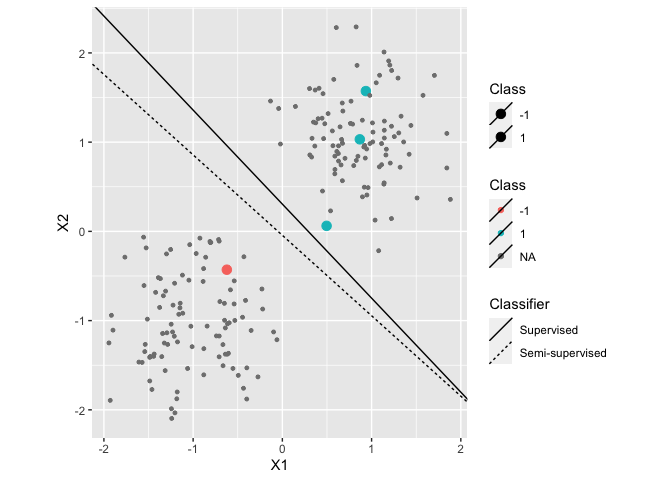# R Semi-Supervised Learning package

This R package provides implementations of several semi-supervised learning methods, in particular, our own work involving constraint based semi-supervised learning.

To cite the package, use either of these two references:

• Krijthe, J. H. (2016). RSSL: R package for Semi-supervised Learning. In B. Kerautret, M. Colom, & P. Monasse (Eds.), Reproducible Research in Pattern Recognition. RRPR 2016. Lecture Notes in Computer Science, vol 10214. (pp. 104–115). Springer International Publishing. https://doi.org/10.1007/978-3-319-56414-2_8. arxiv: https://arxiv.org/abs/1612.07993
• Krijthe, J.H. & Loog, M. (2015). Implicitly Constrained Semi-Supervised Least Squares Classification. In E. Fromont, T. de Bie, & M. van Leeuwen, eds. 14th International Symposium on Advances in Intelligent Data Analysis XIV (Lecture Notes in Computer Science Volume 9385). Saint Etienne. France, pp. 158-169.

# Installation Instructions

This package available on CRAN. The easiest way to install the package is to use:

``install.packages("RSSL")``

To install the latest version of the package using the devtools package:

``````library(devtools)

# Usage

After installation, load the package as usual:

``library(RSSL)``

The following code generates a simple dataset, trains a supervised and two semi-supervised classifiers and evaluates their performance:

``````library(dplyr,warn.conflicts = FALSE)
library(ggplot2,warn.conflicts = FALSE)

set.seed(2)
df <- generate2ClassGaussian(200, d=2, var = 0.2, expected=TRUE)

# Randomly remove labels

# Train classifier
g_nm <- NearestMeanClassifier(Class~.,df,prior=matrix(0.5,2))
g_self <- SelfLearning(Class~.,df,
method=NearestMeanClassifier,
prior=matrix(0.5,2))

# Plot dataset
df %>%
ggplot(aes(x=X1,y=X2,color=Class,size=Class)) +
geom_point() +
coord_equal() +
scale_size_manual(values=c("-1"=3,"1"=3), na.value=1) +
geom_linearclassifier("Supervised"=g_nm,
"Semi-supervised"=g_self)````````````
# Evaluate performance: Squared Loss & Error Rate
mean(loss(g_nm,df))
mean(loss(g_self,df))

mean(predict(g_nm,df)!=df\$Class)
mean(predict(g_self,df)!=df\$Class)``````

# Acknowledgement

Work on this package was supported by Project 23 of the Dutch national program COMMIT.